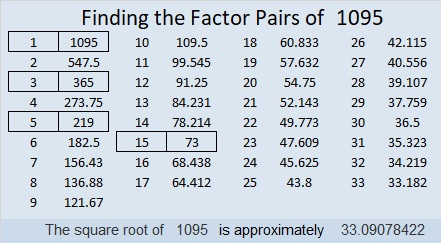# 1095 and Level 1

This awkward-looking puzzle is as simple as clockwork to solve. Put a 5 above the 55 in the top row and another 5 before the 30 in the first column. Then write what number the big hand is pointing to when it is 55 minutes after the hour and so forth until you have written the numbers from 1 to 12 in both the first column and the top row. (You will have to figure out what numbers go with the column and row without clues.)Print the puzzles or type the solution in this excel file: 12 factors 1095-1101

That puzzle had something to do with a clock, while the number 1095 has something to do with the number of days in three non-leap years. Here are some other facts about it:

1 + 0 + 9 + 5 = 15, so 1095 can be evenly divided by 3. Since its last digit is 5, it is also divisible by 5.

• 1095 is a composite number.
• Prime factorization: 1095 = 3 × 5 × 73
• The exponents in the prime factorization are 1, 1, and 1. Adding one to each and multiplying we get (1 + 1)(1 + 1)(1 + 1) = 2 × 2 × 2 = 8. Therefore 1095 has exactly 8 factors.
• Factors of 1095: 1, 3, 5, 15, 73, 219, 365, 1095
• Factor pairs: 1095 = 1 × 1095, 3 × 365, 5 × 219, or 15 × 73
• 1095 has no square factors that allow its square root to be simplified. √1095 ≈ 33.090781095 is the hypotenuse of FOUR Pythagorean triples:
81-1092-1095 which is 3 times (27-364-365)
228-1071-1095 which is 3 times (76-357-365)
657-876-1095 which is (3-4-5) times 219
720-825-1095 which is 15 times (48-55-73)

This site uses Akismet to reduce spam. Learn how your comment data is processed.